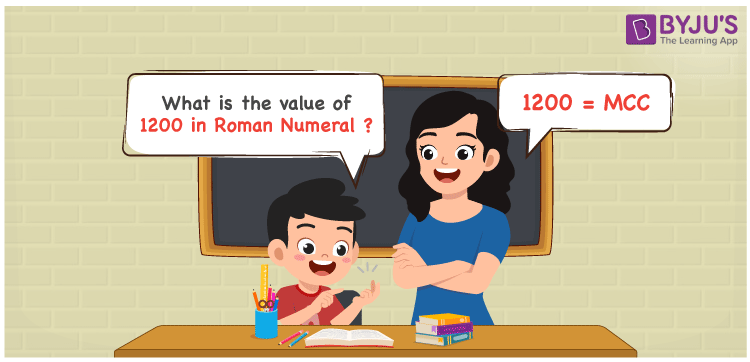# 1200 in Roman Numerals

1200 in Roman Numerals is MCC. Any Roman numeral uses only the 7 primary symbols which are I, V, X, L, C, D, and M, Where M represents 1000 and C represents 100 . What are Roman numerals? can be referred for more details. The details on how to write 1200 are given in the next section of this article.

 Number Roman Numeral 1200 MCC

## How to Write 1200 in Roman Numerals?To convert 1200 in Roman Numerals, we need to represent 1200 as the sum of the fundamental symbols. 1200 can be broken down as the sum of 1000 and 200. Since M is the symbol for 1000, and there is no symbol for 200, 200 can be written as the sum of 100 and 100. Let us understand it numerically;

1200 = 1000 + 200

1200 = 1000 + 100 + 100

1200 = M + C + C

1200 = MCC.

## Video Lesson on Roman Numerals## Frequently Asked Questions on 1200 in Roman Numerals

Q1

### How to write the number 1200 in Roman Numerals?

1200 in Roman Numerals is written as MCC.
Q2

### Do MCC and CCM represent Roman Numerals?

No. MCC represents Roman Numerals and it is the number 1200, whereas CCM is an invalid Roman numeral.
Q3

### What is the year 1200 in Roman numerals?

The year 1200 in Roman Numerals is MCC.